TensorFlow provides tools to have full control of the computations. This is done with the low-level API. On top of that, TensorFlow is equipped with a vast array of APIs to perform many machine learning algorithms. This is the high-level API. TensorFlow calls them estimators

• Low-level API: Build the architecture, optimization of the model from scratch. It is complicated for a beginner
• High-level API: Define the algorithm. It is easer-friendly. TensorFlow provides a toolbox calls estimator to construct, train, evaluate and make a prediction.

In this tutorial, you will use the estimators only. The computations are faster and are easier to implement. The first part of the tutorial explains how to use the gradient descent optimizer to train a linear regression. In a second part, you will use the Boston dataset to predict the price of a house using TensorFlow estimator.

In this tutorial, you will learn:

## How to train a linear regression model

Before we begin to train the model, let's have look at what is a linear regression.

Imagine you have two variables, x and y and your task is to predict the value of knowing the value of . If you plot the data, you can see a positive relationship between your independent variable, x and your dependent variable y.

You may observe, if x=1,y will roughly be equal to 6 and if x=2,y will be around 8.5.

This is not a very accurate method and prone to error, especially with a dataset with hundreds of thousands of points.

A linear regression is evaluated with an equation. The variable y is explained by one or many covariates. In your example, there is only one dependent variable. If you have to write this equation, it will be:

With:

Imagine you fit the model and you find the following solution for:

You can substitute those numbers in the equation and it becomes:

y= 3.8 + 2.78x

You have now a better way to find the values for y. That is, you can replace x with any value you want to predict y. In the image below, we have replace x in the equation with all the values in the dataset and plot the result.

The red line represents the fitted value, that is the values of y for each value of x. You don't need to see the value of x to predict y, for each x there is any which belongs to the red line. You can also predict for values of x higher than 2!

If you want to extend the linear regression to more covariates, you can by adding more variables to the model. The difference between traditional analysis and linear regression is the linear regression looks at how y will react for each variable x taken independently.

Let's see an example. Imagine you want to predict the sales of an ice cream shop. The dataset contains different information such as the weather (i.e rainy, sunny, cloudy), customer informations (i.e salary, gender, marital status).

Traditional analysis will try to predict the sale by let's say computing the average for each variable and try to estimate the sale for different scenarios. It will lead to poor predictions and restrict the analysis to the chosen scenario.

If you use linear regression, you can write this equation:

The algorithm will find the best solution for the weights; it means it will try to minimize the cost (the difference between the fitted line and the data points).

How the algorithm works

The algorithm will choose a random number for each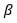and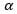and replace the value of x to get the predicted value of y. If the dataset has 100 observations, the algorithm computes 100 predicted values.

We can compute the error, noted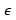of the model, which is the difference between the predicted value and the real value. A positive error means the model underestimates the prediction of y, and a negative error means the model overestimates the prediction of y.

Your goal is to minimize the square of the error. The algorithm computes the mean of the square error. This step is called minimization of the error. For linear regression is the Mean Square Error, also called MSE. Mathematically, it is:

Where:

Note that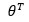means it uses the transpose of the matrices. The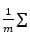is the mathematical notation of the mean.

The goal is to find the best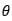that minimize the MSE

If the average error is large, it means the model performs poorly and the weights are not chosen properly. To correct the weights, you need to use an optimizer. The traditional optimizer is called Gradient Descent.

The gradient descent takes the derivative and decreases or increases the weight. If the derivative is positive, the weight is decreased. If the derivative is negative, the weight increases. The model will update the weights and recompute the error. This process is repeated until the error does not change anymore. Each process is called an iteration. Besides, the gradients are multiplied by a learning rate. It indicates the speed of the learning.

If the learning rate is too small, it will take very long time for the algorithm to converge (i.e requires lots of iterations). If the learning rate is too high, the algorithm might never converge.

You can see from the picture above, the model repeats the process about 20 times before to find a stable value for the weights, therefore reaching the lowest error.

Note that, the error is not equal to zero but stabilizes around 5. It means, the model makes a typical error of 5. If you want to reduce the error, you need to add more information to the model such as more variables or use different estimators.

You remember the first equation

The final weights are 3.8 and 2.78. The video below shows you how the gradient descent optimize the loss function to find this weights

## How to train a Linear Regression with TensorFlow

Now that you have a better understanding of what is happening behind the hood, you are ready to use the estimator API provided by TensorFlow to train your first linear regression.

You will use the Boston Dataset, which includes the following variables

crim per capita crime rate by town
zn proportion of residential land zoned for lots over 25,000 sq.ft.
indus proportion of non-retail business acres per town.
nox nitric oxides concentration
rm average number of rooms per dwelling
age proportion of owner-occupied units built before 1940
dis weighted distances to five Boston employment centers
tax full-value property-tax rate per dollars 10,000
ptratio pupil-teacher ratio by town
medv Median value of owner-occupied homes in thousand dollars

You will create three different datasets:

dataset objective shape
Training Train the model and obtain the weights 400, 10
Evaluation Evaluate the performance of the model on unseen data 100, 10
Predict Use the model to predict house value on new data 6, 10

The objectives is to use the features of the dataset to predict the value of the house.

During the second part of the tutorial, you will learn how to use TensorFlow with three different way to import the data:

• With Pandas
• With Numpy
• Only TF

Note that, all options provide the same results.

You will learn how to use the high-level API to build, train an evaluate a linear regression model. If you were using the low-level API, you had to define by hand the:

• Loss function
• Matrices multiplication
• Graph and tensor

This is tedious and more complicated for beginner.

## Pandas

You need to import the necessary libraries to train the model.

```import pandas as pd
from sklearn import datasets
import tensorflow as tf
import itertools			```

Step 1) Import the data with panda.

You define the column names and store it in COLUMNS. You can use pd.read_csv() to import the data.

```COLUMNS = ["crim", "zn", "indus", "nox", "rm", "age",
"dis", "tax", "ptratio", "medv"]```

You can print the shape of the data.

`print(training_set.shape, test_set.shape, prediction_set.shape)			`

Output

`(400, 10) (100, 10) (6, 10)`

Note that the label, i.e. your y, is included in the dataset. So you need to define two other lists. One containing only the features and one with the name of the label only. These two lists will tell your estimator what are the features in the dataset and what column name is the label

It is done with the code below.

```FEATURES = ["crim", "zn", "indus", "nox", "rm",
"age", "dis", "tax", "ptratio"]
LABEL = "medv"			```

Step 2) Convert the data

You need to convert the numeric variables in the proper format. Tensorflow provides a method to convert continuous variable: tf.feature_column.numeric_column().

In the previous step, you define a list a feature you want to include in the model. Now you can use this list to convert them into numeric data. If you want to exclude features in your model, feel free to drop one or more variables in the list FEATURES before you construct the feature_cols

Note that you will use Python list comprehension with the list FEATURES to create a new list named feature_cols. It helps you avoid writing nine times tf.feature_column.numeric_column(). A list comprehension is a faster and cleaner way to create new lists

`feature_cols = [tf.feature_column.numeric_column(k) for k in FEATURES]			`

Step 3) Define the estimator

In this step, you need to define the estimator. Tensorflow currently provides 6 pre-built estimators, including 3 for classification task and 3 for regression task:

• Regressor
• DNNRegressor
• LinearRegressor
• DNNLineaCombinedRegressor
• Classifier
• DNNClassifier
• LinearClassifier
• DNNLineaCombinedClassifier

In this tutorial, you will use the Linear Regressor. To access this function, you need to use tf.estimator.

The function needs two arguments:

• feature_columns: Contains the variables to include in the model
• model_dir: path to store the graph, save the model parameters, etc

Tensorflow will automatically create a file named train in your working directory. You need to use this path to access the Tensorboard.

```estimator = tf.estimator.LinearRegressor(
feature_columns=feature_cols,
model_dir="train")			```

Output

```INFO:tensorflow:Using default config.
INFO:tensorflow:Using config: {'_model_dir': 'train', '_tf_random_seed': None, '_save_summary_steps': 100, '_save_checkpoints_steps': None, '_save_checkpoints_secs': 600, '_session_config': None, '_keep_checkpoint_max': 5, '_keep_checkpoint_every_n_hours': 10000, '_log_step_count_steps': 100, '_train_distribute': None, '_service': None, '_cluster_spec': <tensorflow.python.training.server_lib.ClusterSpec object at 0x1a215dc550>, '_task_type': 'worker', '_task_id': 0, '_global_id_in_cluster': 0, '_master': '', '_evaluation_master': '', '_is_chief': True, '_num_ps_replicas': 0, '_num_worker_replicas': 1}```

The tricky part with TensorFlow is the way to feed the model. Tensorflow is designed to work with parallel computing and very large dataset. Due to the limitation of the machine resources, it is impossible to feed the model with all the data at once. For that, you need to feed a batch of data each time. Note that, we are talking about huge dataset with millions or more records. If you don't add batch, you will end up with a memory error.

For instance, if your data contains 100 observations and you define a batch size of 10, it means the model will see 10 observations for each iteration (10*10).

When the model has seen all the data, it finishes one epoch. An epoch defines how many times you want the model to see the data. It is better to set this step to none and let the model performs iteration number of time.

A second information to add is if you want to shuffle the data before each iteration. During the training, it is important to shuffle the data so that the model does not learn specific pattern of the dataset. If the model learns the details of the underlying pattern of the data, it will have difficulties to generalize the prediction for unseen data. This is called overfitting. The model performs well on the training data but cannot predict correctly for unseen data.

TensorFlow makes this two steps easy to do. When the data goes to the pipeline, it knows how many observations it needs (batch) and if it has to shuffle the data.

To instruct Tensorflow how to feed the model, you can use pandas_input_fn. This object needs 5 parameters:

• x: feature data
• y: label data
• batch_size: batch. By default 128
• num_epoch: Number of epoch, by default 1
• shuffle: Shuffle or not the data. By default, None

You need to feed the model many times so you define a function to repeat this process. all this function get_input_fn.

```def get_input_fn(data_set, num_epochs=None, n_batch = 128, shuffle=True):
return tf.estimator.inputs.pandas_input_fn(
x=pd.DataFrame({k: data_set[k].values for k in FEATURES}),
y = pd.Series(data_set[LABEL].values),
batch_size=n_batch,
num_epochs=num_epochs,
shuffle=shuffle)			```

The usual method to evaluate the performance of a model is to:

• Train the model
• Evaluate the model in a different dataset
• Make prediction

Tensorflow estimator provides three different functions to carry out this three steps easily.

Step 4): Train the model

You can use the estimator train to evaluate the model. The train estimator needs an input_fn and a number of steps. You can use the function you created above to feed the model. Then, you instruct the model to iterate 1000 times. Note that, you don't specify the number of epochs, you let the model iterates 1000 times. If you set the number of epoch to 1, then the model will iterate 4 times: There are 400 records in the training set, and the batch size is 128

1. 128 rows
2. 128 rows
3. 128 rows
4. 16 rows

Therefore, it is easier to set the number of epoch to none and define the number of iteration.

```estimator.train(input_fn=get_input_fn(training_set,
num_epochs=None,
n_batch = 128,
shuffle=False),
steps=1000)			```

Output

```INFO:tensorflow:Calling model_fn.
INFO:tensorflow:Done calling model_fn.
INFO:tensorflow:Create CheckpointSaverHook.
INFO:tensorflow:Graph was finalized.
INFO:tensorflow:Running local_init_op.
INFO:tensorflow:Done running local_init_op.
INFO:tensorflow:Saving checkpoints for 1 into train/model.ckpt.
INFO:tensorflow:loss = 83729.64, step = 1
INFO:tensorflow:global_step/sec: 238.616
INFO:tensorflow:loss = 13909.657, step = 101 (0.420 sec)
INFO:tensorflow:global_step/sec: 314.293
INFO:tensorflow:loss = 12881.449, step = 201 (0.320 sec)
INFO:tensorflow:global_step/sec: 303.863
INFO:tensorflow:loss = 12391.541, step = 301 (0.327 sec)
INFO:tensorflow:global_step/sec: 308.782
INFO:tensorflow:loss = 12050.5625, step = 401 (0.326 sec)
INFO:tensorflow:global_step/sec: 244.969
INFO:tensorflow:loss = 11766.134, step = 501 (0.407 sec)
INFO:tensorflow:global_step/sec: 155.966
INFO:tensorflow:loss = 11509.922, step = 601 (0.641 sec)
INFO:tensorflow:global_step/sec: 263.256
INFO:tensorflow:loss = 11272.889, step = 701 (0.379 sec)
INFO:tensorflow:global_step/sec: 254.112
INFO:tensorflow:loss = 11051.9795, step = 801 (0.396 sec)
INFO:tensorflow:global_step/sec: 292.405
INFO:tensorflow:loss = 10845.855, step = 901 (0.341 sec)
INFO:tensorflow:Saving checkpoints for 1000 into train/model.ckpt.
INFO:tensorflow:Loss for final step: 5925.9873.
```

You can check the Tensorboard will the following command:

```activate hello-tf
# For MacOS
tensorboard --logdir=./train
# For Windows
tensorboard --logdir=train			```

You can evaluate the fit of your model on the test set with the code below:

```ev = estimator.evaluate(
input_fn=get_input_fn(test_set,
num_epochs=1,
n_batch = 128,
shuffle=False))			```

Output

```INFO:tensorflow:Calling model_fn.
INFO:tensorflow:Done calling model_fn.
INFO:tensorflow:Starting evaluation at 2018-05-13-01:43:13
INFO:tensorflow:Graph was finalized.
INFO:tensorflow:Restoring parameters from train/model.ckpt-1000
INFO:tensorflow:Running local_init_op.
INFO:tensorflow:Done running local_init_op.
INFO:tensorflow:Finished evaluation at 2018-05-13-01:43:13
INFO:tensorflow:Saving dict for global step 1000: average_loss = 32.15896, global_step = 1000, loss = 3215.896
```

You can print the loss with the code below:

```loss_score = ev["loss"]
print("Loss: {0:f}".format(loss_score))			```

Output

`Loss: 3215.895996`

The model has a loss of 3215. You can check the summary statistic to get an idea of how big the error is.

`training_set['medv'].describe()`

Output

```count    400.000000
mean      22.625500
std        9.572593
min        5.000000
25%       16.600000
50%       21.400000
75%       25.025000
max       50.000000
Name: medv, dtype: float64			```

From the summary statistic above, you know that the average price for a house is 22 thousand, with a minimum price of 9 thousands and maximum of 50 thousand. The model makes a typical error of 3k dollars.

Step 6) Make the prediction

Finally, you can use the estimator predict to estimate the value of 6 Boston houses.

```y = estimator.predict(
input_fn=get_input_fn(prediction_set,
num_epochs=1,
n_batch = 128,
shuffle=False))			```

To print the estimated values of , you can use this code:

`predictions = list(p["predictions"] for p in itertools.islice(y, 6))print("Predictions: {}".format(str(predictions)))			`

Output

```INFO:tensorflow:Calling model_fn.
INFO:tensorflow:Done calling model_fn.
INFO:tensorflow:Graph was finalized.
INFO:tensorflow:Restoring parameters from train/model.ckpt-1000
INFO:tensorflow:Running local_init_op.
INFO:tensorflow:Done running local_init_op.
Predictions: [array([32.297546], dtype=float32), array([18.96125], dtype=float32), array([27.270979], dtype=float32), array([29.299236], dtype=float32), array([16.436684], dtype=float32), array([21.460876], dtype=float32)]
```

The model forecast the following values:

House Prediction 1 32.29 2 18.96 3 27.27 4 29.29 5 16.43 7 21.46

Note that we don't know the true value of . In the tutorial of deep learning, you will try to beat the linear model

## Numpy Solution

This section explains how to train the model using a numpy estimator to feed the data. The method is the same exept that you will use numpy_input_fn estimator.

Step 1) Import the data

First of all, you need to differentiate the feature variables from the label. You need to do this for the training data and evaluation. It is faster to define a function to split the data.

```def prepare_data(df):
X_train = df[:, :-3]
y_train = df[:,-3]
return X_train, y_train			```

You can use the function to split the label from the features of the train/evaluate dataset

```X_train, y_train = prepare_data(training_set_n)
X_test, y_test = prepare_data(test_set_n)			```

You need to exclude the last column of the prediction dataset because it contains only NaN

`x_predict = prediction_set_n[:, :-2]			`

Confirm the shape of the array. Note that, the label should not have a dimension, it means (400,).

`print(X_train.shape, y_train.shape, x_predict.shape)			`

Output

`(400, 9) (400,) (6, 9)			`

You can construct the feature columns as follow:

`feature_columns = [      tf.feature_column.numeric_column('x', shape=X_train.shape[1:])]			`

The estimator is defined as before, you instruct the feature columns and where to save the graph.

```estimator = tf.estimator.LinearRegressor(
feature_columns=feature_columns,
model_dir="train1")			```

Output

```INFO:tensorflow:Using default config.
INFO:tensorflow:Using config: {'_model_dir': 'train1', '_tf_random_seed': None, '_save_summary_steps': 100, '_save_checkpoints_steps': None, '_save_checkpoints_secs': 600, '_session_config': None, '_keep_checkpoint_max': 5, '_keep_checkpoint_every_n_hours': 10000, '_log_step_count_steps': 100, '_train_distribute': None, '_service': None, '_cluster_spec': <tensorflow.python.training.server_lib.ClusterSpec object at 0x1a218d8f28>, '_task_type': 'worker', '_task_id': 0, '_global_id_in_cluster': 0, '_master': '', '_evaluation_master': '', '_is_chief': True, '_num_ps_replicas': 0, '_num_worker_replicas': 1}			```

You can use the numpy estimapor to feed the data to the model and then train the model. Note that, we define the input_fn function before to ease the readability.

```# Train the estimatortrain_input = tf.estimator.inputs.numpy_input_fn(
x={"x": X_train},
y=y_train,
batch_size=128,
shuffle=False,
num_epochs=None)
estimator.train(input_fn = train_input,steps=5000) 			```

Output

```INFO:tensorflow:Calling model_fn.
INFO:tensorflow:Done calling model_fn.
INFO:tensorflow:Create CheckpointSaverHook.
INFO:tensorflow:Graph was finalized.
INFO:tensorflow:Running local_init_op.
INFO:tensorflow:Done running local_init_op.
INFO:tensorflow:Saving checkpoints for 1 into train1/model.ckpt.
INFO:tensorflow:loss = 83729.64, step = 1
INFO:tensorflow:global_step/sec: 490.057
INFO:tensorflow:loss = 13909.656, step = 101 (0.206 sec)
INFO:tensorflow:global_step/sec: 788.986
INFO:tensorflow:loss = 12881.45, step = 201 (0.126 sec)
INFO:tensorflow:global_step/sec: 736.339
INFO:tensorflow:loss = 12391.541, step = 301 (0.136 sec)
INFO:tensorflow:global_step/sec: 383.305
INFO:tensorflow:loss = 12050.561, step = 401 (0.260 sec)
INFO:tensorflow:global_step/sec: 859.832
INFO:tensorflow:loss = 11766.133, step = 501 (0.117 sec)
INFO:tensorflow:global_step/sec: 804.394
INFO:tensorflow:loss = 11509.918, step = 601 (0.125 sec)
INFO:tensorflow:global_step/sec: 753.059
INFO:tensorflow:loss = 11272.891, step = 701 (0.134 sec)
INFO:tensorflow:global_step/sec: 402.165
INFO:tensorflow:loss = 11051.979, step = 801 (0.248 sec)
INFO:tensorflow:global_step/sec: 344.022
INFO:tensorflow:loss = 10845.854, step = 901 (0.288 sec)
INFO:tensorflow:Saving checkpoints for 1000 into train1/model.ckpt.
INFO:tensorflow:Loss for final step: 5925.985.
Out:
<tensorflow.python.estimator.canned.linear.LinearRegressor at 0x1a1b6ea860>
```

You replicate the same step with a different estimator to evaluate your model

```eval_input = tf.estimator.inputs.numpy_input_fn(
x={"x": X_test},
y=y_test,
shuffle=False,
batch_size=128,
num_epochs=1)
estimator.evaluate(eval_input,steps=None) 			```

Output

```INFO:tensorflow:Calling model_fn.
INFO:tensorflow:Done calling model_fn.
INFO:tensorflow:Starting evaluation at 2018-05-13-01:44:00
INFO:tensorflow:Graph was finalized.
INFO:tensorflow:Restoring parameters from train1/model.ckpt-1000
INFO:tensorflow:Running local_init_op.
INFO:tensorflow:Done running local_init_op.
INFO:tensorflow:Finished evaluation at 2018-05-13-01:44:00
INFO:tensorflow:Saving dict for global step 1000: average_loss = 32.158947, global_step = 1000, loss = 3215.8945
Out:
{'average_loss': 32.158947, 'global_step': 1000, 'loss': 3215.8945}
```

Finaly, you can compute the prediction. It should be the similar as pandas.

```test_input = tf.estimator.inputs.numpy_input_fn(
x={"x": x_predict},
batch_size=128,
num_epochs=1,
shuffle=False)
y = estimator.predict(test_input)
predictions = list(p["predictions"] for p in itertools.islice(y, 6))
print("Predictions: {}".format(str(predictions)))			```

Output

```INFO:tensorflow:Calling model_fn.
INFO:tensorflow:Done calling model_fn.
INFO:tensorflow:Graph was finalized.
INFO:tensorflow:Restoring parameters from train1/model.ckpt-1000
INFO:tensorflow:Running local_init_op.
INFO:tensorflow:Done running local_init_op.
Predictions: [array([32.297546], dtype=float32), array([18.961248], dtype=float32), array([27.270979], dtype=float32), array([29.299242], dtype=float32), array([16.43668], dtype=float32), array([21.460878], dtype=float32)]
```

## Tensorflow solution

The last section is dedicated to a TensorFlow solution. This method is sligthly more complicated than the other one.

Note that if you use Jupyter notebook, you need to Restart and clean the kernel to run this session.

TensorFlow has built a great tool to pass the data into the pipeline. In this section, you will build the input_fn function by yourself.

Step 1) Define the path and the format of the data

First of all, you declare two variables with the path of the csv file. Note that, you have two files, one for the training set and one for the testing set.

`import tensorflow as tf`
`df_train = "E:/boston_train.csv"`
`df_eval = "E:/boston_test.csv"`

Then, you need to define the columns you want to use from the csv file. We will use all. After that, you need to declare the type of variable it is.

Floats variable are defined by [0.]

```COLUMNS = ["crim", "zn", "indus", "nox", "rm", "age",
"dis", "tax", "ptratio", "medv"]RECORDS_ALL = [[0.0], [0.0], [0.0], [0.0],[0.0],[0.0],[0.0],[0.0],[0.0],[0.0]]			```

Step 2) Define the input_fn function

The function can be broken into three part:

1. Import the data
2. Create the iterator
3. Consume the data

Below is the overal code to define the function. The code will be explained after

```def input_fn(data_file, batch_size, num_epoch = None):
# Step 1
def parse_csv(value):
columns = tf.decode_csv(value, record_defaults= RECORDS_ALL)
features = dict(zip(COLUMNS, columns))
#labels = features.pop('median_house_value')
labels =  features.pop('medv')
return features, labels

# Extract lines from input files using the
Dataset API.    dataset = (tf.data.TextLineDataset(data_file) # Read text file
.map(parse_csv))

dataset = dataset.repeat(num_epoch)
dataset = dataset.batch(batch_size)
# Step 3
iterator = dataset.make_one_shot_iterator()
features, labels = iterator.get_next()
return features, labels			```

** Import the data**

For a csv file, the dataset method reads one line at a time. To build the dataset, you need to use the object TextLineDataset. Your dataset has a header so you need to use skip(1) to skip the first line. At this point, you only read the data and exclude the header in the pipeline. To feed the model, you need to separate the features from the label. The method used to apply any transformation to the data is map.

This method calls a function that you will create in order to instruct how to transform the data.In a nutshell, you need to pass the data in the TextLineDataset object, exclude the header and apply a transformation which is instructed by a function.Code explanation

• tf.data.TextLineDataset(data_file): This line read the csv file
• .skip(1) : skip the header
• .map(parse_csv)): parse the records into the tensorsYou need to define a function to instruct the map object. You can call this function parse_csv.

This function parses the csv file with the method tf.decode_csv and declares the features and the label. The features can be declared as a dictionary or a tuple. You use the dictionary method because it is more convenient.Code explanation

• tf.decode_csv(value, record_defaults= RECORDS_ALL): the method decode_csv uses the output of the TextLineDataset to read the csv file. record_defaults instructs TensorFlow about the columns type.
• dict(zip(_CSV_COLUMNS, columns)): Populate the dictionary with all the columns extracted during this data processing
• features.pop('median_house_value'): Exclude the target variable from the feature variable and create a label variable

The Dataset needs further elements to iteratively feeds the Tensors. Indeed, you need to add the method repeat to allow the dataset to continue indefinitely to feed the model. If you don't add the method, the model will iterate only one time and then throw an error because no more data are fed in the pipeline.

After that, you can control the batch size with the batch method. It means you tell the dataset how many data you want to pass in the pipeline for each iteration. If you set a big batch size, the model will be slow.

Step 3) Create the iterator

Now you are ready for the second step: create an iterator to return the elements in the dataset.

The simplest way of creating an operator is with the method make_one_shot_iterator.

After that, you can create the features and labels from the iterator.

Step 4) Consume the data

You can check what happens with input_fn function. You need to call the function in a session to consume the data. You try with a batch size equals to 1.

Note that, it prints the features in a dictionary and the label as an array.

It will show the first line of the csv file. You can try to run this code many times with different batch size.

```next_batch = input_fn(df_train, batch_size = 1, num_epoch = None)
with tf.Session() as sess:
first_batch  = sess.run(next_batch)
print(first_batch)			```

Output

`({'crim': array([2.3004], dtype=float32), 'zn': array([0.], dtype=float32), 'indus': array([19.58], dtype=float32), 'nox': array([0.605], dtype=float32), 'rm': array([6.319], dtype=float32), 'age': array([96.1], dtype=float32), 'dis': array([2.1], dtype=float32), 'tax': array([403.], dtype=float32), 'ptratio': array([14.7], dtype=float32)}, array([23.8], dtype=float32))`

Step 4) Define the feature column

You need to define the numeric columns as follow:

```X1= tf.feature_column.numeric_column('crim')
X2= tf.feature_column.numeric_column('zn')
X3= tf.feature_column.numeric_column('indus')
X4= tf.feature_column.numeric_column('nox')
X5= tf.feature_column.numeric_column('rm')
X6= tf.feature_column.numeric_column('age')
X7= tf.feature_column.numeric_column('dis')
X8= tf.feature_column.numeric_column('tax')
X9= tf.feature_column.numeric_column('ptratio')			```

Note that you need to combined all the variables in a bucket

`base_columns = [X1, X2, X3,X4, X5, X6,X7, X8, X9]			`

Step 5) Build the model

You can train the model with the estimator LinearRegressor.

`model = tf.estimator.LinearRegressor(feature_columns=base_columns, model_dir='train3')			`

Output

`INFO:tensorflow:Using default config. INFO:tensorflow:Using config: {'_model_dir': 'train3', '_tf_random_seed': None, '_save_summary_steps': 100, '_save_checkpoints_steps': None, '_save_checkpoints_secs': 600, '_session_config': None, '_keep_checkpoint_max': 5, '_keep_checkpoint_every_n_hours': 10000, '_log_step_count_steps': 100, '_train_distribute': None, '_service': None, '_cluster_spec': <tensorflow.python.training.server_lib.ClusterSpec object at 0x1820a010f0>, '_task_type': 'worker', '_task_id': 0, '_global_id_in_cluster': 0, '_master': '', '_evaluation_master': '', '_is_chief': True, '_num_ps_replicas': 0, '_num_worker_replicas': 1}`

You need to use a lambda function to allow to write the argument in the function inpu_fn. If you don't use a lambda function, you cannot train the model.

```# Train the estimatormodel.train(steps =1000,
input_fn= lambda : input_fn(df_train,batch_size=128, num_epoch = None))			```

Output

```INFO:tensorflow:Calling model_fn.
INFO:tensorflow:Done calling model_fn.
INFO:tensorflow:Create CheckpointSaverHook.
INFO:tensorflow:Graph was finalized.
INFO:tensorflow:Running local_init_op.
INFO:tensorflow:Done running local_init_op.
INFO:tensorflow:Saving checkpoints for 1 into train3/model.ckpt.
INFO:tensorflow:loss = 83729.64, step = 1
INFO:tensorflow:global_step/sec: 72.5646
INFO:tensorflow:loss = 13909.657, step = 101 (1.380 sec)
INFO:tensorflow:global_step/sec: 101.355
INFO:tensorflow:loss = 12881.449, step = 201 (0.986 sec)
INFO:tensorflow:global_step/sec: 109.293
INFO:tensorflow:loss = 12391.541, step = 301 (0.915 sec)
INFO:tensorflow:global_step/sec: 102.235
INFO:tensorflow:loss = 12050.5625, step = 401 (0.978 sec)
INFO:tensorflow:global_step/sec: 104.656
INFO:tensorflow:loss = 11766.134, step = 501 (0.956 sec)
INFO:tensorflow:global_step/sec: 106.697
INFO:tensorflow:loss = 11509.922, step = 601 (0.938 sec)
INFO:tensorflow:global_step/sec: 118.454
INFO:tensorflow:loss = 11272.889, step = 701 (0.844 sec)
INFO:tensorflow:global_step/sec: 114.947
INFO:tensorflow:loss = 11051.9795, step = 801 (0.870 sec)
INFO:tensorflow:global_step/sec: 111.484
INFO:tensorflow:loss = 10845.855, step = 901 (0.897 sec)
INFO:tensorflow:Saving checkpoints for 1000 into train3/model.ckpt.
INFO:tensorflow:Loss for final step: 5925.9873.
Out:
<tensorflow.python.estimator.canned.linear.LinearRegressor at 0x18225eb8d0>
```

You can evaluate the fit of you model on the test set with the code below:

```results = model.evaluate(steps =None,input_fn=lambda: input_fn(df_eval, batch_size =128, num_epoch = 1))
for key in results:
print("   {}, was: {}".format(key, results[key]))			```

Output

```INFO:tensorflow:Calling model_fn.
INFO:tensorflow:Done calling model_fn.
INFO:tensorflow:Starting evaluation at 2018-05-13-02:06:02
INFO:tensorflow:Graph was finalized.
INFO:tensorflow:Restoring parameters from train3/model.ckpt-1000
INFO:tensorflow:Running local_init_op.
INFO:tensorflow:Done running local_init_op.
INFO:tensorflow:Finished evaluation at 2018-05-13-02:06:02
INFO:tensorflow:Saving dict for global step 1000: average_loss = 32.15896, global_step = 1000, loss = 3215.896
average_loss, was: 32.158958435058594
loss, was: 3215.89599609375
global_step, was: 1000
```

The last step is predicting the value of based on the value of , the matrices of the features. You can write a dictionary with the values you want to predict. Your model has 9 features so you need to provide a value for each. The model will provide a prediction for each of them.

In the code below, you wrote the values of each features that is contained in the df_predict csv file.

You need to write a new input_fn function because there is no label in the dataset. You can use the API from_tensor from the Dataset.

```prediction_input = {
'crim': [0.03359,5.09017,0.12650,0.05515,8.15174,0.24522],
'zn': [75.0,0.0,25.0,33.0,0.0,0.0],
'indus': [2.95,18.10,5.13,2.18,18.10,9.90],
'nox': [0.428,0.713,0.453,0.472,0.700,0.544],
'rm': [7.024,6.297,6.762,7.236,5.390,5.782],
'age': [15.8,91.8,43.4,41.1,98.9,71.7],
'dis': [5.4011,2.3682,7.9809,4.0220,1.7281,4.0317],
'tax': [252,666,284,222,666,304],
'ptratio': [18.3,20.2,19.7,18.4,20.2,18.4]
}
def test_input_fn():
dataset = tf.data.Dataset.from_tensors(prediction_input)
return dataset

# Predict all our prediction_inputpred_results = model.predict(input_fn=test_input_fn)			```

Finaly, you print the predictions.

```for pred in enumerate(pred_results):
print(pred)			```

Output

```INFO:tensorflow:Calling model_fn.
INFO:tensorflow:Done calling model_fn.
INFO:tensorflow:Graph was finalized.
INFO:tensorflow:Restoring parameters from train3/model.ckpt-1000
INFO:tensorflow:Running local_init_op.
INFO:tensorflow:Done running local_init_op.
(0, {'predictions': array([32.297546], dtype=float32)})
(1, {'predictions': array([18.96125], dtype=float32)})
(2, {'predictions': array([27.270979], dtype=float32)})
(3, {'predictions': array([29.299236], dtype=float32)})
(4, {'predictions': array([16.436684], dtype=float32)})
(5, {'predictions': array([21.460876], dtype=float32)})

INFO:tensorflow:Calling model_fn. INFO:tensorflow:Done calling model_fn. INFO:tensorflow:Graph was finalized. INFO:tensorflow:Restoring parameters from train3/model.ckpt-5000 INFO:tensorflow:Running local_init_op. INFO:tensorflow:Done running local_init_op. (0, {'predictions': array([35.60663], dtype=float32)}) (1, {'predictions': array([22.298521], dtype=float32)}) (2, {'predictions': array([25.74533], dtype=float32)}) (3, {'predictions': array([35.126694], dtype=float32)}) (4, {'predictions': array([17.94416], dtype=float32)}) (5, {'predictions': array([22.606628], dtype=float32)})```

Summary

To train a model, you need to:

• Define the features: Independent variables: X
• Define the label: Dependent variable: y
• Construct a train/test set
• Define the initial weight
• Define the loss function: MSE
• Optimize the model: Gradient descent
• Define:
• Learning rate
• Number of epoch
• Batch size

In this tutorial, you learned how to use the high level API for a linear regression estimator. You need to define:

1. Feature columns. If continuous: tf.feature_column.numeric_column(). You can populate a list with python list comprehension
2. The estimator: tf.estimator.LinearRegressor(feature_columns, model_dir)
3. A function to import the data, the batch size and epoch: input_fn()

After that, you are ready to train, evaluate and make prediction with train(), evaluate() and predict()

YOU MIGHT LIKE: